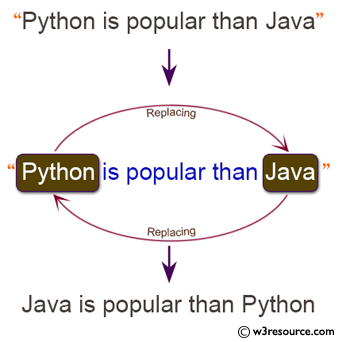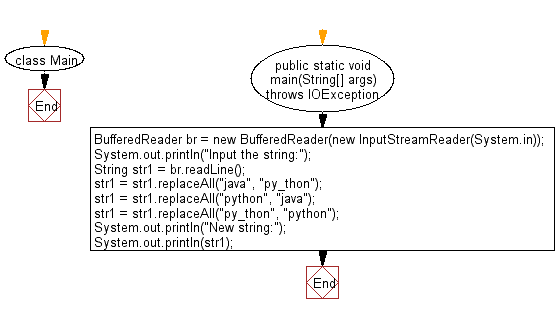# Java Exercises: Replace a string "python" with "java" and "java" with "python" in a given string

## Java Basic: Exercise-230 with Solution

Write a Java program to replace a string "python" with "java" and "java" with "python" in a given string.

Input:

English letters (including single byte alphanumeric characters, blanks, symbols) are given on one line. The length of the input character string is 1000 or less.

Pictorial Presentation:Sample Solution:

Java Code:

`````` import java.io.IOException;

class Main {
public static void main(String[] args) throws IOException {
System.out.println("Input the string:");

str1 = str1.replaceAll("java", "py_thon");
str1 = str1.replaceAll("python", "java");
str1 = str1.replaceAll("py_thon", "python");
System.out.println("New string:");
System.out.println(str1);
}
}

```
```

Sample Output:

```Input the string:
python is more popular than java
New string:
java is more popular than python
```

Flowchart:Java Code Editor:

What is the difficulty level of this exercise?

Test your Programming skills with w3resource's quiz.

﻿

## Java: Tips of the Day

Java: Anagrams

Generates all anagrams of a string.

```public static List<String> anagrams(String input) {
if (input.length() <= 2) {
return input.length() == 2
? Arrays.asList(input, input.substring(1) + input.substring(0, 1))
: Collections.singletonList(input);
}
return IntStream.range(0, input.length())
.mapToObj(i -> new SimpleEntry<>(i, input.substring(i, i + 1)))
.flatMap(entry ->
anagrams(input.substring(0, entry.getKey()) + input.substring(entry.getKey() + 1))
.stream()
.map(s -> entry.getValue() + s))
.collect(Collectors.toList());
}
```